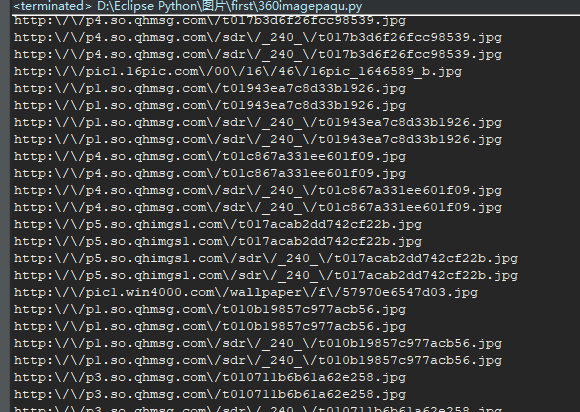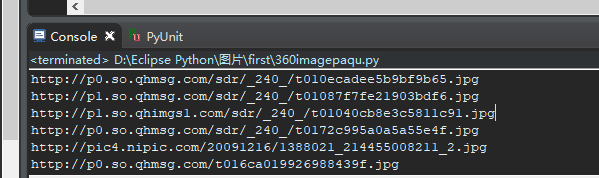# 爬取多态加载图片同时保存到本地指定文件夹

url = "http://image.so.com/i?src=360pic_strong&z=1&i=0&cmg=1e3ddcecc474bf6d0d486ca671c63d47&q=%E7%BE%8E%E5%A5%B3%E5%9B%BE%E7%89%87"
html = requests.get(url).text
#print html
soup = BeautifulSoup(content,"html.parser")

clist = re.findall('http:\\\/\\\/p.*?\.jpg',html)dic={}
for i in clist:
if i in dic.keys():
dic[i]+=1
else:
dic[i]=1

name_list=[]
num_list=[]
for key in dic:
name_list.append(key)
num_list.append(dic[key])


 b.append(name_list[i].replace("\/","/"))urllib.urlretrieve(b[t],'C:\\Users\\ASUS\\Desktop\\txt\\img/%s.jpg' % x)

# coding=utf-8
import urllib
import requests
from bs4 import BeautifulSoup
import re

url = "http://image.so.com/i?src=360pic_strong&z=1&i=0&cmg=1e3ddcecc474bf6d0d486ca671c63d47&q=%E7%BE%8E%E5%A5%B3%E5%9B%BE%E7%89%87"
html = requests.get(url).text
#print html
soup = BeautifulSoup(content,"html.parser")
#print soup
clist = re.findall('http:\\\/\\\/p.*?\.jpg',html)

dic={}
for i in clist:
if i in dic.keys():
dic[i]+=1
else:
dic[i]=1

name_list=[]
num_list=[]
for key in dic:
name_list.append(key)
num_list.append(dic[key])
#print (name_list)
b = []
i=1
while i<len(name_list):
b.append(name_list[i].replace("\/","/"))
i=i+1
for i in b:
print i
t=0
x=0
while t<len(b):
urllib.urlretrieve(b[t],'C:\\Users\\ASUS\\Desktop\\txt\\img/%s.jpg' % x)
t=t+1
x=x+1
print "正在爬取第"+str(x)+"张图片"
print "爬取完成"